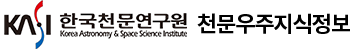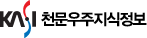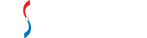통합검색

통합검색

인기검색어논문 상세보기

• 홈으로 이동
TOWARD A NEXT GENERATION SOLAR CORONAGRAPH: DIFFRACTED LIGHT SIMULATION AND TEST RESULTS FOR A CONE OCCULTER WITH TAPERED SURFACE
• 저자
Yang, Heesu
• 저널명
The Korean Astronomical Society
• 발행년도
2018
• 권호
V .51, N .2
• 원문보기
In a solar coronagraph, the most important component is an occulter to block the direct light from the disk of the sun. Because the intensity of the solar outer corona is $10^{-6}$ to $10^{-10}$ times of that of the solar disk ( $I_{\odot}$ ), it is necessary to minimize scattering at the optical elements and diffraction at the occulter. Using a Fourier optic simulation and a stray light test, we investigated the performance of a compact coronagraph that uses an external truncated-cone occulter without an internal occulter and Lyot stop. In the simulation, the diffracted light was minimized to the order of $7.6{\times}10^{-10}I_{\odot}$ when the cone angle ${\theta}_c$ was about $0.39^{\circ}$ . The performance of the cone occulter was then tested by experiment. The level of the diffracted light reached the order of $6{\times}10^{-9}I_{\odot}$ at ${\theta}_c=0.40^{\circ}$ . This is sufficient to observe the outer corona without additional optical elements such as a Lyot stop or inner occulter. We also found the manufacturing tolerance of the cone angle to be $0.05^{\circ}$ , the lateral alignment tolerance was $45{\mu}m$ , and the angular alignment tolerance was $0.043^{\circ}$ . Our results suggest that the physical size of coronagraphs can be shortened significantly by using a cone occulter.